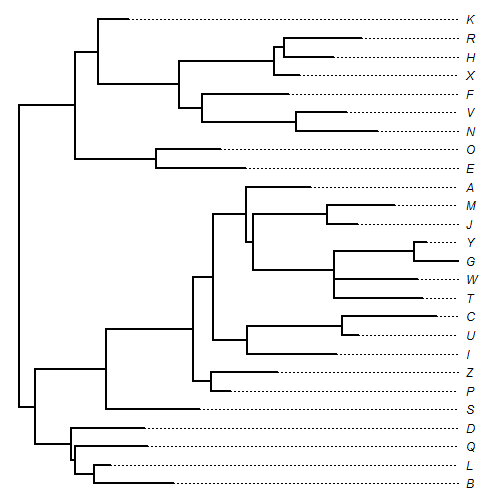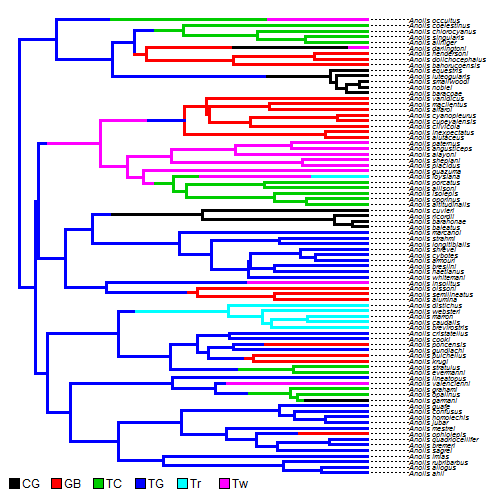## Monday, May 8, 2017

### Plotting a non-ultrametric tree with dotted lines to aligned tip labels

phytools already has an internal tree plotting function that uses dotted lines to connect the tips of non-ultrametric trees to aligned tip labels. This function can be seen, for instance, in the phytools method `plot.cophylo` (e.g., here).

However, it is also easy to script essentially the same functionality using `plotTree` or `plotSimmap` internally. For example:

``````library(phytools)
tree
``````
``````##
## Phylogenetic tree with 26 tips and 25 internal nodes.
##
## Tip labels:
##  B, L, Q, D, S, P, ...
##
## Rooted; includes branch lengths.
``````
``````par(fg="transparent")
plotTree(cw)
obj<-get("last_plot.phylo",envir=.PlotPhyloEnv)
par(fg="black")
text(rep(max(obj\$xx[1:Ntip(cw)]),Ntip(cw)),obj\$yy[1:Ntip(cw)],
labels=cw\$tip.label,font=3,pos=4)
for(i in 1:Ntip(cw)) lines(c(obj\$xx[i],max(obj\$xx[1:Ntip(cw)])),
rep(obj\$yy[i],2),lty="dotted")
``````In this case, the tip alignment (& dotted lines) are based on the highest end-point of any edge in the tree. For ultrametric trees, we can also add a further offset if desired. For instance:

``````data(anoletree)
plotTree(anoletree,fsize=0.6,ftype="i",ylim=c(-1,Ntip(anoletree)),
plot=FALSE)
``````
``````obj<-get("last_plot.phylo",envir=.PlotPhyloEnv)
xlim<-obj\$x.lim
ylim<-obj\$y.lim
offset<-0.1*diff(xlim)
xlim<-xlim+offset
plot.new()
plot.window(xlim=xlim,ylim=ylim)
cw<-reorder(anoletree)
text(rep(max(obj\$xx[1:Ntip(cw)])+offset,Ntip(cw)),obj\$yy[1:Ntip(cw)],
labels=gsub("_"," ",cw\$tip.label),font=3,pos=4,cex=0.6,offset=0.02)
for(i in 1:Ntip(cw)) lines(c(obj\$xx[i],max(obj\$xx[1:Ntip(cw)])+offset),
rep(obj\$yy[i],2),lty="dotted")
par(fg="transparent")
``````
``````## no colors provided. using the following legend:
##        CG        GB        TC        TG        Tr        Tw
##   "black"     "red"  "green3"    "blue"    "cyan" "magenta"
``````
``````par(fg="black")